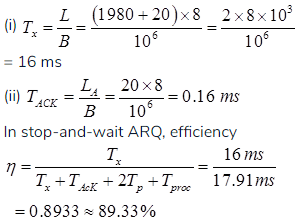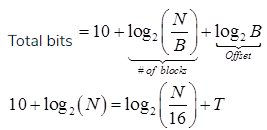# GATE Solved Paper 2017-19 - GATE 2017 Shift 1

>>>>>>>>GATE 2017 Shift 1

• A

75• B

76• C

72• D

74• Option : B
• Explanation :
A miss is not considered a conflict miss if the block is accessed for the first time.
1st round: (2+2) misses
2nd round: (4+4) misses
∴Total = 4 + (8 × 9) = 76 conflict misses

• A

2• B

1• C

4• D

3• Option : A
• Explanation :The given expression is (a-1) * ((( b + c ) / 3 ) + d)
The optimal generated code is:
Load R1, b       R1 ⟵ b
Load R2, c       R2 ⟵ c
ADD R1, R2      R1 ⟵ R1 + R2
Div R1, 3       R1 ⟵ R1/3
Load R2, d       R2 ⟵ d
Add R1, R2       R1 ⟵ R1 + R2
Load R2, a       R2 ⟵ a
Sub R2, 1       R2 ⟵ R2 - 1
Mul R2, R1       R2 ⟵ R2 * R1
Hence minimum 2 registers required.

• A

89.33• B

98.33• C

94.11• D

95.11• Option : A
• Explanation :
Given Data:
B = 1Mbps
Tproc = 0.25ms
Tp = 0.75ms
L = 1980 Bytes
LOH = 20 Bytes
LA = 20 Bytes
Efficiency (η) = ?• A

14• B

13• C

12• D

10• Option : A
• Explanation :where T is the required length of TAG field
∴ T = 14

 CR Student Name Course Name SA CA SA CB SA CC SB CB SB CC SC CA SC CB SC CC SD CA SD CB SD CC SD CD SE CD SE CA SE CB SF CA SF CB SF CC
The following query is made on the database.
T1 ← πCourseNameStudentName=’SA’(CR))
T2 ← CR ÷ T1
The number of rows in T2 is ________.

Note – Numerical Type question

• A

2• B

3• C

4• D

5• Option : C
• Explanation :
Explanation: Result of T1:
CA
CB
CC

Result of T2 = CR/T1
SA
SC
SD
SF
Total = 4
Therefore, option C is correct

Alternate Solution :
The output of query T1 will be the courses enrolled by student SA i.e CA, CB, CC
The output of query T2 will be the names of the students who enroll all the courses that are the output of query T1 (division operator) i.e CA, CB, CC
From the table we can see that SA, SC, SD, SF have enrolled all the courses so the output of T2 will have 4 row
Related Quiz.
GATE 2017 Shift 1